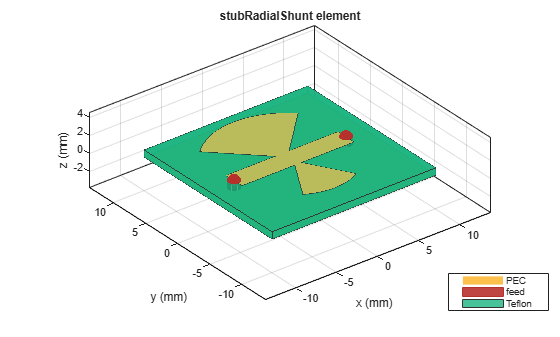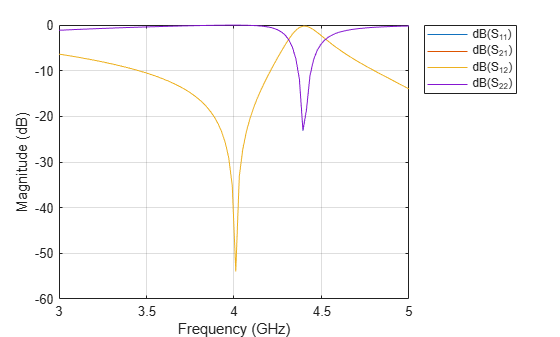# dielectric

Dielectric material for use as substrate

## Syntax

``d = dielectric(material)``
``d = dielectric(Name=Value)``

## Description

example

````d = dielectric(material)` returns dielectric materials for use as a substrate in PCB components. ```
````d = dielectric(Name=Value)` returns dielectric materials based on the properties specified by one or more `Name,Value` pair arguments.```

## Examples

collapse all

Create shunt radial stub of type double.

```stub = stubRadialShunt(StubType='double'); stub.OuterRadius = [0.0085 0.0065]; stub.InnerRadius = [0.0012 0.0008]; stub.Angle = [90 60];```

`show(stub)`Plot s-parameters.

```spar = sparameters(stub,linspace(3e9,5e9,50)); rfplot(spar)```## Input Arguments

collapse all

Material from the dielectric catalog, specified as one of the values from the `DielectricCatalog`.

Example: `'FR4'`

Data Types: `char`

### Name-Value Arguments

Specify optional pairs of arguments as `Name1=Value1,...,NameN=ValueN`, where `Name` is the argument name and `Value` is the corresponding value. Name-value arguments must appear after other arguments, but the order of the pairs does not matter.

Example: `Name=Air`

Name of the dielectric material you want to specify in the output, specified as a character vector.

Example: `Name='Taconic_TLC'`

Data Types: `char`

Relative permittivity of the dielectric material, specified as a real-valued vector.

Example: `EpsilonR=4.8000`

Data Types: `double`

Loss in the dielectric material, specified as a vector.

Example: `LossTangent=0.0260`

Data Types: `double`

Thickness of the dielectric material in meters along default z-axis, specified as a positive vector. This property applies only when you call the function with no output arguments.

Example: `Thickness=0.05`

Data Types: `double`

## Output Arguments

collapse all

Dielectric material, returned as an object handle. You can use the dielectric material object handle to add dielectric material as a substrate to any PCB component.

## Version History

Introduced in R2016a